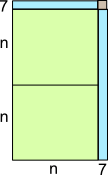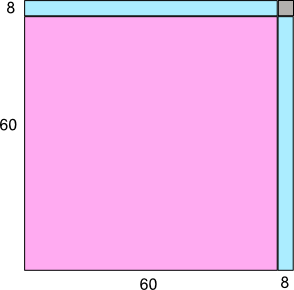# Connecting multiplication in arithmetic to algebra and area patterns

## Overview

Area or grid models are used to represent two digit multiplication in arithmetic, multiplication of binomials, represent polynomials, and area models for squares.

I went to the bakery to get some cookies and found a shelf with discounted bakery items. On the shelf were eighteen containers of cookies with twenty-three cookies in each. Each was labeled two dozen cookies with a big X over the two dozen and 23 written above it. I guess they didn't want to sell them and take a chance of disappointing a customer.
I bought all 18 containers so how many cookies did I buy?

## Setting up a representation

Draw a visual to represent the containers and cookies.Or a person could simplify it without the cookies and outline 18 columns for containers with 23 rows for cookies.1. The 18 columns represent the containers.
2. The 23 rows represent the 23 cookies in each container.

## Solving the problem with an algorithm

Okay, If we think of solving the problem with a nice number strategy, then we could change the problem from 18 containers of 23 cookies.

To 20 containers of 23 cookies. This would make it easy to double 23 to 46 and multiply by ten to get 460 cookies.

Then subtract two groups of 23 cookies that aren't needed as there are only 18 containers. Or 460 cookies - 46 cookies = 414 cookies.

Okay.

How can an algorithm be invented?

## Solving the problem with an algorithm

When we multiple two two digit numbers we must know, or discover, all four digits need to be involved in a way that is different from addition and subtraction.

This idea is inferred by the fact that when 12 * 3 is multiplied, the three must multiply 10 and 2.

(10 * 3 + 2 * 3)

Unlike addition where the three is only added with the two: 12 + 3; 15.

Continue this logic for our problem (23 * 18).

Decompose both numbers into: 20 + 3 and 10 + 8 and multiply all four numbers.

 Two tens & three ones 2 3 One ten & eight ones 1 8

Eight times three   8 * 3   =   2 4
Eight times twenty   8 * 2 0 = 1 6 0
Tens time three 1 0 * 3   =   3 0
Ten times twenty 1 0 * 2 0 = 2 0 0

##### 4

However.

Why?

What does all this mean?

Let's review what was multiplied and how each is represented in rows and columns with an area representation.

• Ten times twenty is represented by blue.
• Eight times twenty is represented yellow.
• Ten times three is represented green.
• Eight times three is represented purple.## OKAY ... Let's generalize what was multiplied:

(20 + 3) * (10 + 8)
23 * 18 =

Multiply all values for all places:

 First factor 20 + 3 Second factor 10 + 8

 First digit from the first factor (3) times the first digit of the second factor (8) 8 * 3 = 24 First digit from the first factor (8) times the second digit of the second factor (2 with a value of 20) 8 * 20 = 160 Second digit from the first factor times (1 with a value of 10) the first digit of the second factor (3) 10 * 3 = 30 Second digit from the first factor (1 with a value of 10) times the second digit of the second factor (2 with a value of 20) 10 * 20 = 200 184 + 230 + 414

## Here it is for binomials!

### Rule for multiplication of binomials

(X + Y) * (X + Y)

• First digit from the first factor times the first digit of the second factor
(First - F)
• First digit from the first factor times the second digit of the second factor
(Outside - O)
• Second digit from the first factor times the first digit of the second factor
• (Inside - I)
• Second digit from the first factor times the second digit of the second factor
(Last - L)
 First factor x + y Second factor x + y

 First digit from the first factor times the first digit of the second factor (F) x * x = x2 First digit from the first factor times the second digit of the second factor (O) x * y = xy Second digit from the first factor times the first digit of the second factor (I) x * y = xy Second digit from the first factor times the second digit of the second factor (L) y * y = y2 -- - --- - -- x2 + 2xy + y2See why elementary teachers who teach beyond algorithmic use are important for the development of their learners?

Brain buster: Try 122

• 122
• (10 + 2)2
• (10 + 2)(10 + 2)
• 102 + 2*2*10 + 22
• 100 + 40 + 4
• 144

Sweet!

Make some of your own or try some examples below.

Enjoy!